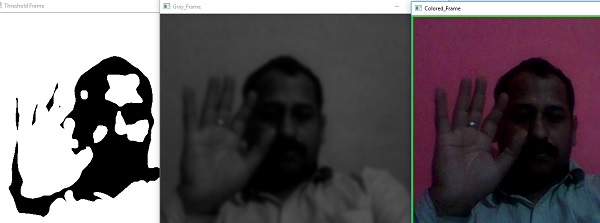# WebCam Motion Detector program in Python ?

In this we are going to write python program which is going to analyse the images taken from the webcam and try to detect the movement and store the time-interval of the webcam video in a csv file.

## Required Library

We are going to use the OpenCV & pandas library for that. If it’s not already installed, you can install it using pip, with something like:

\$pip install opencv2, pandas

## Example Code

#Import required libraries
import cv2
import pandas as pd
import time
from datetime import datetime

#Initialise variables
stillImage = None
motionImage = [ None, None ]
time = []

# Initializing the DataFrame with start and end time
df = pd.DataFrame(columns = ["start", "end"])

# Capturing video
video = cv2.VideoCapture(0)

while True:
# Start reading image from video
motion = 0

# Convert color image to gray_scale image
gray = cv2.cvtColor(frame, cv2.COLOR_BGR2GRAY)
gray = cv2.GaussianBlur(gray, (21, 21), 0)
if stillImage is None:
stillImage = gray
continue
# Still Image and current image.
diff_frame = cv2.absdiff(stillImage, gray)

# change the image to white if static background and current frame is greater than 25.
thresh_frame = cv2.threshold(diff_frame, 25, 255, cv2.THRESH_BINARY)
thresh_frame = cv2.dilate(thresh_frame, None, iterations = 2)
# Finding contour and hierarchy from a moving object.
contours,hierachy = cv2.findContours(thresh_frame.copy(),
cv2.RETR_EXTERNAL, cv2.CHAIN_APPROX_SIMPLE)
for contour in contours:
if cv2.contourArea(contour) < 10000:
continue
motion = 1
(x, y, w, h) = cv2.boundingRect(contour)
cv2.rectangle(frame, (x, y), (x + w, y + h), (0, 255, 0), 3)
# Append current status of motion
motionImage.append(motion)
motionImage = motionImage[-2:]
# Append Start time of motion
if motionImage[-1] == 1 and motionImage[-2] == 0:
time.append(datetime.now())

# Append End time of motion
if motionImage[-1] == 0 and motionImage[-2] == 1:
time.append(datetime.now())
# Displaying image in gray_scale
cv2.imshow("Gray_Frame", gray)

# Display black and white frame & if the intensity difference is > 25, it turns white
cv2.imshow("Threshold Frame", thresh_frame)
# Display colored frame
cv2.imshow("Colored_Frame", frame)

key = cv2.waitKey(1)
# Press q to stop the process
if key == ord('q'):
if motion == 1:
time.append(datetime.now())
break

# Append time of motion
for i in range(0, len(time), 2):
df = df.append({"Start":time[i], "End":time[i + 1]}, ignore_index = True)

# Creating a csv file in which time of movements will be saved
df.to_csv("FrameInMotion_time.csv")

video.release()

# close window
cv2.destroyAllWindows()

## OutputWe can see we’ll get 3 different Windows which are going to display our current movement from webcam in three different modes (grayscale, colored & black&White).

It will also store the datetime of our webcam Motion in a csv and our output from csv will be something like:

### FrameMotion_time.csv (output)

start     end      End                            Start
0                  2019-02-21 18:10:59.718005     2019-02-21 18:08:35.791487